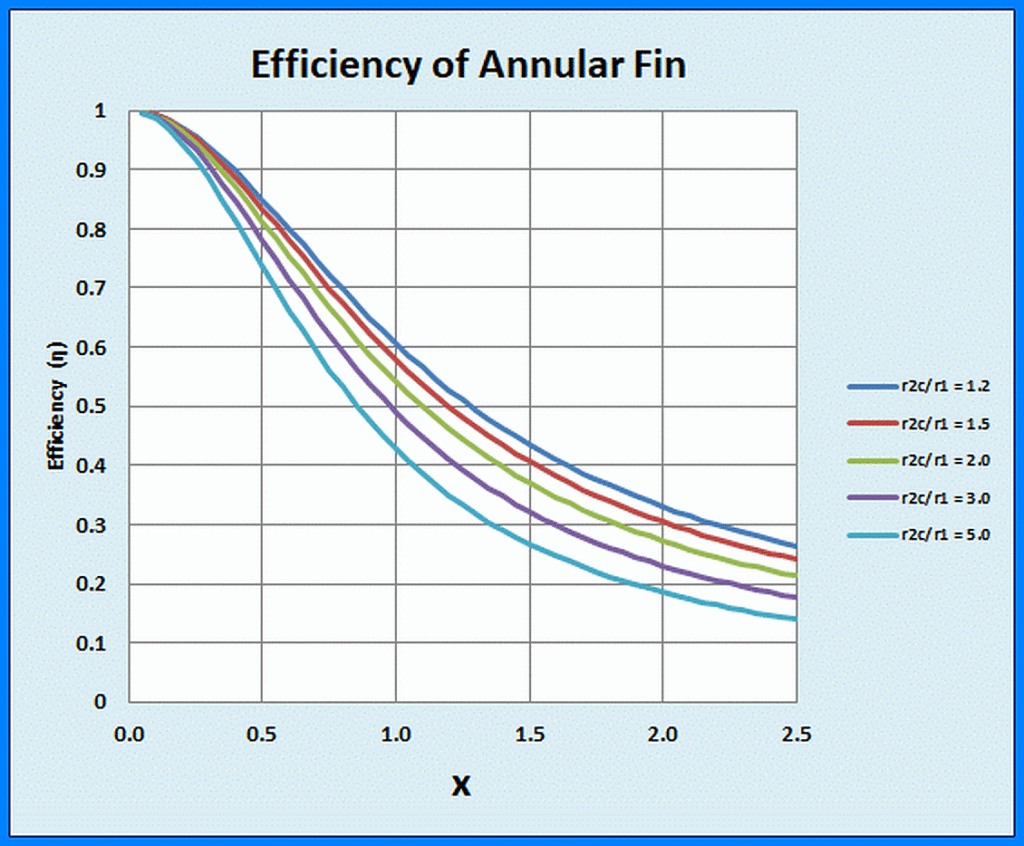# Analytical Solution for Efficiency of Straight, Annular Fins

UPDATED: 08/28/2022

This workbook evaluates the efficiency (qactual/qmax) of straight annular fins based on the analytical solution of the governing heat balance equation.   Excel’s intrinsic Bessel functions are invoked in user-supplied VBA functions.   The calculation and the display are only of the “bottom line,” the efficiency of a single annular fin as a function of the fin parameter and the radius ratio (fin tip radius/fin root radius).Efficiency of straight annular fins computed from the analytical solution. Efficiency (= q/qmax) is plotted for five values of the radius ratio (router/rinner) as a function of the fin parameter

This workbook was developed to verify solutions given by the more general Extended Surface module, The HTTextnd module displays the entire temperature profile in this fin geometry and a myriad of others in addition to the fin efficiency and effectiveness.   Photos of a variety of extended surface configurations, including the straight, annular fin, may be viewed here.